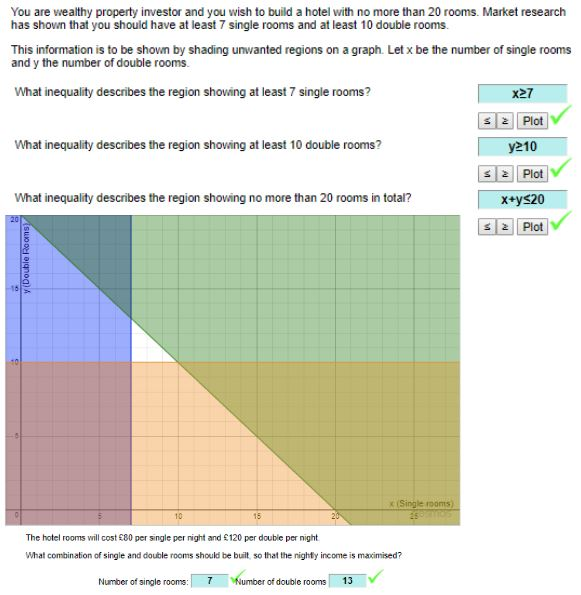# Linear Programming

…………………………………………………………..
From Transum, try Linear Programming which provides a really easy way for teachers to illustrate several Linear Programming problems. Students could try these as an online activity.I am pleased to see that a reminder of Inequalities is the first activity, something I always start with when teaching Linear Programming. Levels 1 to 5 provide 5 different problems providing very typical linear programming problems and we also have Exam Style Questions. The very generous Free model on Transum allows access to the extensive library of resources including checking answers such as illustrated here;  a subscription additionally provides answers to all of the pupil online exercises such as Exam Questions, quizzes and puzzles as well as additional benefits.

Students are required not just to solve these probems but to interpret solutions to such problems. For Linear Programming there are some excellent ways of using technology to help  learning. We can look at online utilities from zweigmedia and the use of the Excel Solver.

This example from MEI (MEI Decision 1 Legacy Specimen Paper) will be used to illustrate the use of these tools.

A vet is treating a farm animal. He must provide minimum daily requirements of an antibiotic, a vitamin and a nutrient. He has two types of medicine available, tablets and liquid. The table summarises what the medicines contain and the requirements.The tablets cost £0.38 each and liquid medicine costs £1 per dose.
The vet wants to find the cheapest way to treat the animal.
We have x as the number of tablets which the vet prescribes per day, and y is the number of doses of liquid medicine, giving us the linear programming problem:
Minimise    0.38x + y
Subject to
3x +   2y   ≥ 18
2x +   4y   ≥ 16
10x + 50y ≥ 100

Firstly we will look at zweigmedia’s Linear Programming Grapher. This is intuitive to use. Note the option to see examples. Fraction mode is selected here.
Selecting Solve gives a clear solution illustrated graphically.We see that the optimal solution, if x and y can take any values, is a cost of £3.20,
with x = 20/3 and y = 2/3.We could also solve the problem using the Simplex Tool. As well as a solution it is possible to see the simplex tableaus. The Simplex Tool is needed for any problems with more than two variables.If we wish to restrict x and y to integers, we can turn to Excel and use Solver. This allows a choice of making x and y integer or not.

Using the same example, we can set up the problem in Excel.
Vet Problem with Excel Solver is the Excel spreadsheet with annotation to explain the answer report and for full instructions on setting up the spreadsheet, use this file:
Solver Excel to solve LP problems – Colleen Youngand use Solver..We could choose to restrict x and y to integers with Solver.giving us the solution:For a further example, this time a maximisation problem from Edexcel have a look at this Excel file, Badges LP Edexcel. Each sheet in the workbook is fully annotated to explain the method.A version of this post is also available for students on Mathematics for Students. Note the excellent notes and examples on Linear Programming available from CIMT, one of a series of chapters on Discrete Mathematics.